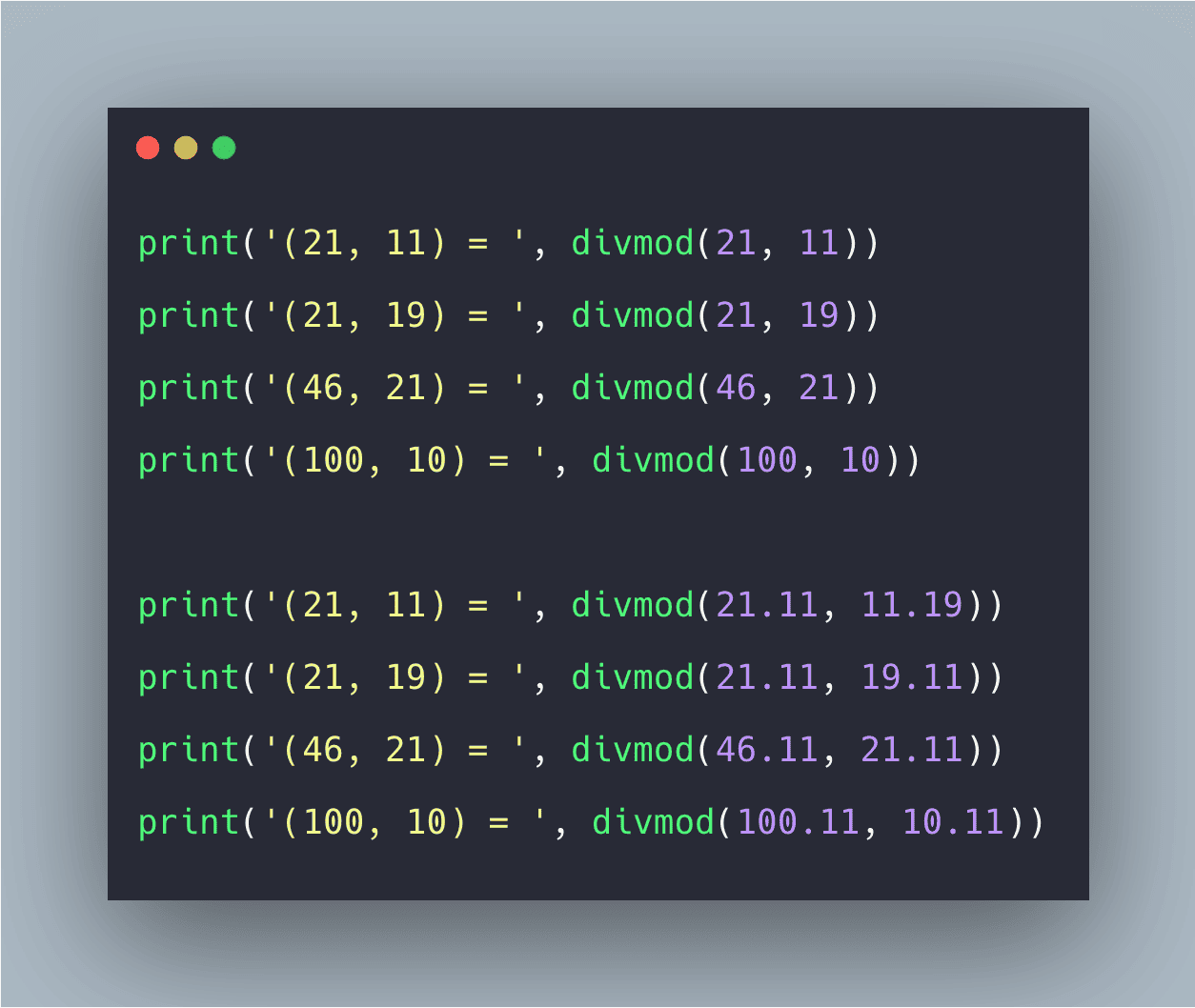# Python divmod() Function: The Complete Guide

0
5The divmod() method takes two parameters, x and y, where x is treated as numerator and y are treated as the denominator.

## Algorithm

1. Initialize the new variable, say a with the given integer and a variable counter to 0.
2. Run a loop till the given integer becomes 0 and keep decrementing it.
3. Save the value returned by divmod(n, a) in two variables, say x and y.
4. Check if y is 0, this will imply that n is perfectly divisible by a, incrementing the counter value.
5. Check if the counter value is greater than 2; if yes, the number is not prime, else it is prime.
`divmod(divident, divisor)`

### Arguments

Divident is the number that you want to divide.

The divisor is the number that you want to divide with.

### Example

See the following code example.

```# app.py

print('(21, 11) = ', divmod(21, 11))
print('(21, 19) = ', divmod(21, 19))
print('(46, 21) = ', divmod(46, 21))
print('(100, 10) = ', divmod(100, 10))```

See the following output.

```➜  pyt python3 app.py
(21, 11) =  (1, 10)
(21, 19) =  (1, 2)
(46, 21) =  (2, 4)
(100, 10) =  (10, 0)
➜  pyt```

Let’s take the float values and see the output.

```# app.py

print('(21, 11) = ', divmod(21.11, 11.19))
print('(21, 19) = ', divmod(21.11, 19.11))
print('(46, 21) = ', divmod(46.11, 21.11))
print('(100, 10) = ', divmod(100.11, 10.11))```

See the output.

```➜  pyt python3 app.py
(21, 11) =  (1.0, 9.92)
(21, 19) =  (1.0, 2.0)
(46, 21) =  (2.0, 3.8900000000000006)
(100, 10) =  (9.0, 9.120000000000005)
➜  pyt```

## Errors And Exceptions

1. If either of the arguments says a and b, is the float data type, the result is (q, a%b). So here, q is the whole part of the quotient.
2. If the second argument is 0, it returns Zero Division Error.
3. If the first argument is 0, it returns (0, 0).

That’s it for this tutorial.

This site uses Akismet to reduce spam. Learn how your comment data is processed.